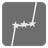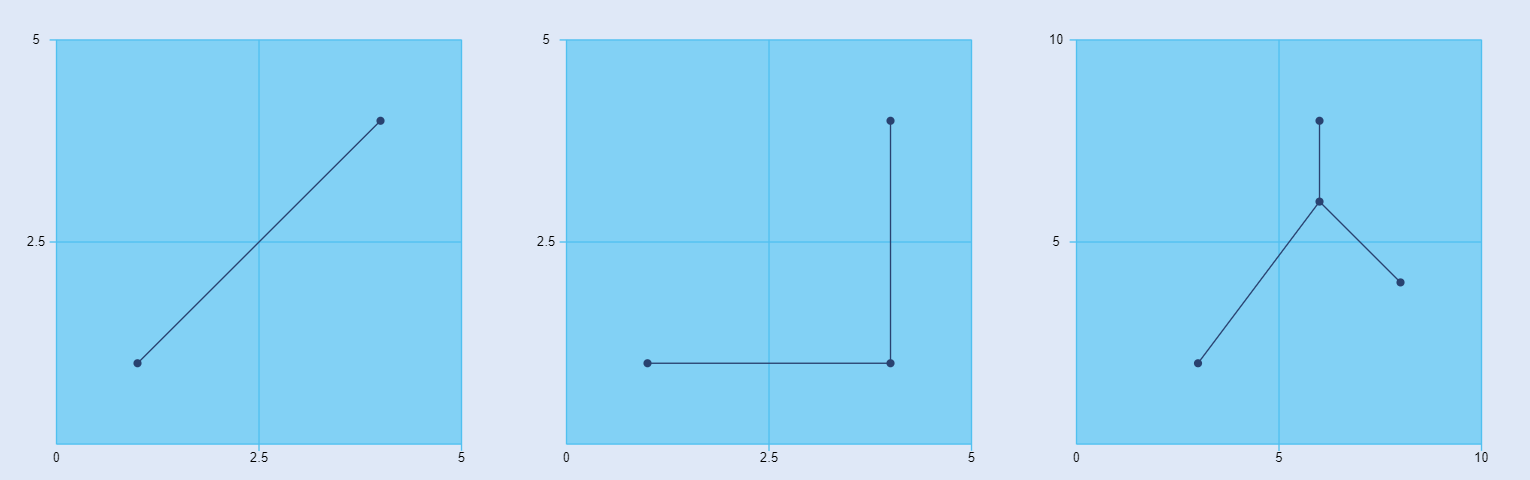Connect Stars
Undefined
English JA

In this mission you'll have to make the Minimum spanning tree (Wikipedia) .

You are given a list of coordinates of vertices. Each coordinate is a tuple of x (integer) and y (integer).
You have to return a list (or an iterable) of lines that connect all vertices. The total length of lines should be the minimum. Each line is a tuple of two integers. These integers represent the index of list of input.

NOTE:

• The output of tests has a unique combination of lines.

Example:

```connect_stars([(1, 1), (4, 4)])) == [(0, 1)]                         # or [(1, 0)]
connect_stars([(1, 1), (4, 1), (4, 4)])) == [(0, 1), (1, 2)]         # or [(1, 2), (0, 1)] , [(1, 0), (2,1)] etc
connect_stars([(6, 6), (6, 8), (8, 4), (3, 2)])) == [(0, 1), (0, 2), (0, 3)]   # or [(2, 0), (0, 3), (1, 0)] etc
```Input:

• A list of coordinates of vertices.
• Each coordinate is a tuple of x (integer) and y (integer).

Output:

• A list (or an iterable) of lines.
• Each line is a tuple of two integers. These integers represent the index of list of input.
• The order of lines and each index is arbitrary (see examples).

Precondition:

• 2 ≤ len(input) ≤ 50
• 0 ≤ x (,y) ≤ 999

Settings
Code:
CheckiO Extensions

CheckiO Extensions allow you to use local files to solve missions. More info in a blog post.

In order to install CheckiO client you'll need installed Python (version at least 3.8)

Install CheckiO Client first:

`pip3 install checkio_client`

`checkio --domain=py config --key=`

Sync solutions into your local folder

`checkio sync`

(in beta testing) Launch local server so your browser can use it and sync solution between local file end extension on the fly. (doesn't work for safari)

`checkio serv -d`

Alternatevly, you can install Chrome extension or FF addon

`checkio install-plugin`
`checkio install-plugin --ff`
`checkio install-plugin --chromium`

Read more here about other functionality that the checkio client provides. Feel free to submit an issue in case of any difficulties.

Sync...